# Quiz 7: Digital Communication

The maximum information rate on the channel is restricted and limited by so many factors. Hartley's Law : According to Hartley's Law the rate of information on the channel depends on type of the coding used for data, time taken to transmit the data down the channel and available channel bandwidth.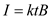Here,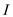is the amount of information to be sent in bits per second.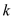is the constant whose value is dependent on type of coding.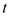time taken to send the information in second.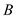available channel bandwidth in Hz From this relation if the available channel bandwidth is less than the time taken to send the data is more. Shannon-Hartley's Theorem : According to Shannon-Hartley theorem the maximum data rate down the channel is dependent on number of levels used for a signal digitization to transmit and the channel bandwidth.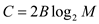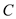is the channel capacity for data transmissionis available channel bandwidth in Hz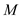is number of levels used for digitization. From this relation if the number of levels for digitization is increased then the maximum channel capacity for more information is increased for a particular channel. Similarly from the Shannon limit principle the maximum channel data rate also depends on signal-to-noise ratio presented at the receiver during signal transmission.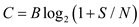is the channel capacity for data transmissionis available channel bandwidth in Hz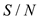is signal-to-noise ratio. From the relation higher the signal-to-noise ratio if more comfort for high data rate. Therefore, the factors channel bandwidth, number of levels for signal digitization, signal coding, time to transmit the data and signal-to-noise ratio are going to limit the information rate down the channel. Because for a particular channel the bandwidth is fixed, if number of levels are increased it is less immune to noise, depending on available channel bandwidth the time taken to transmit the data is restricted and similarly the practical digital equipments restrict the signal-to-noise ratio. Hence all these factors are going to limit the information rate.
Sampling is a technique in which an analog signal is converted into digital form. In this technique a band limited analog signal is sampled at regular interval of time and these samples can be recovered and the original analog signal is retrieved at the receiver. The sampling of analog signal and its signal recovery at the receiver is shown in Figure 1.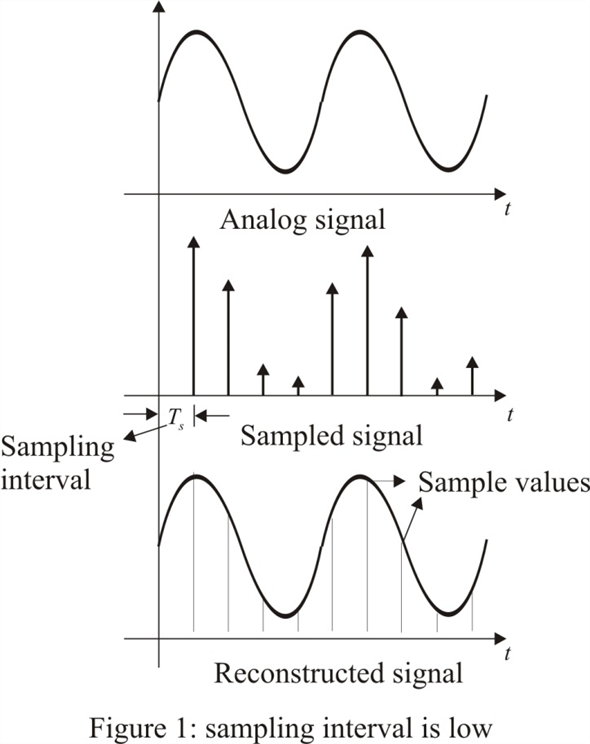Here the sampling interval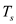is high and is dependent of signal maximum frequency. Therefore, for efficient sampling and signal recovery the sampling frequency must be greater than or eqaul to the twice of the maximum frequency of the message signal. Efficient sampling process in frequency domain is illustrated in Figure 2.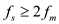Here,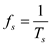is the sampling frequency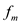is the maximum signal frequency.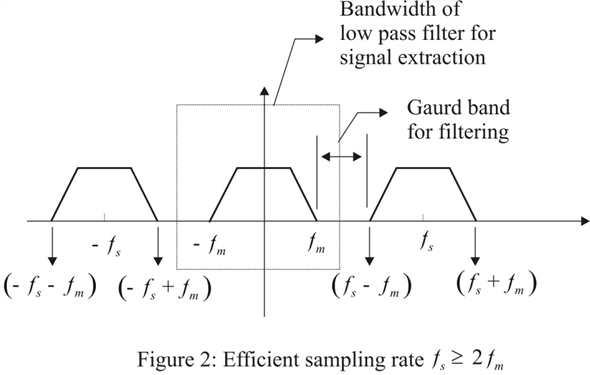In Figure 2, the original signal (spectrum) centered at origin can be retrieved from its sampled signal (from the entire spectrum) by low pass filtering that utilizes the guard band for efficient filtering in order to safe retrieval of original message signal. Therefore if there exist a guard band in the spectrum then it gives a provision to low pass filter to adjust its cutoff frequency to extract the original signal without any of its frequency components. Suppose, unfortunately, if the sampling frequency is less than the twice of the maximum frequency then retrieval of original signal from its sampled signal is not possible since there exist no guard band or at least sharp edge cutoff frequency in between side band signal and the signal centered at origin as shown in Figure 3.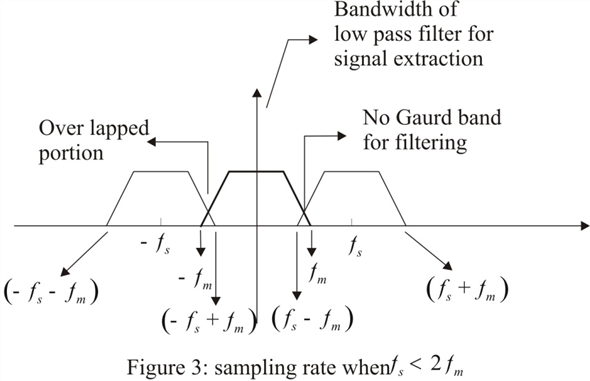From Figure 3, as there is no guard band since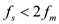it is not at all possible to extract the original signal from its sampled signal. Therefore, for efficient sampling always maintain a guard band in between successive signal spectrums otherwise the signal cannot be filtered out by the low pass filter.